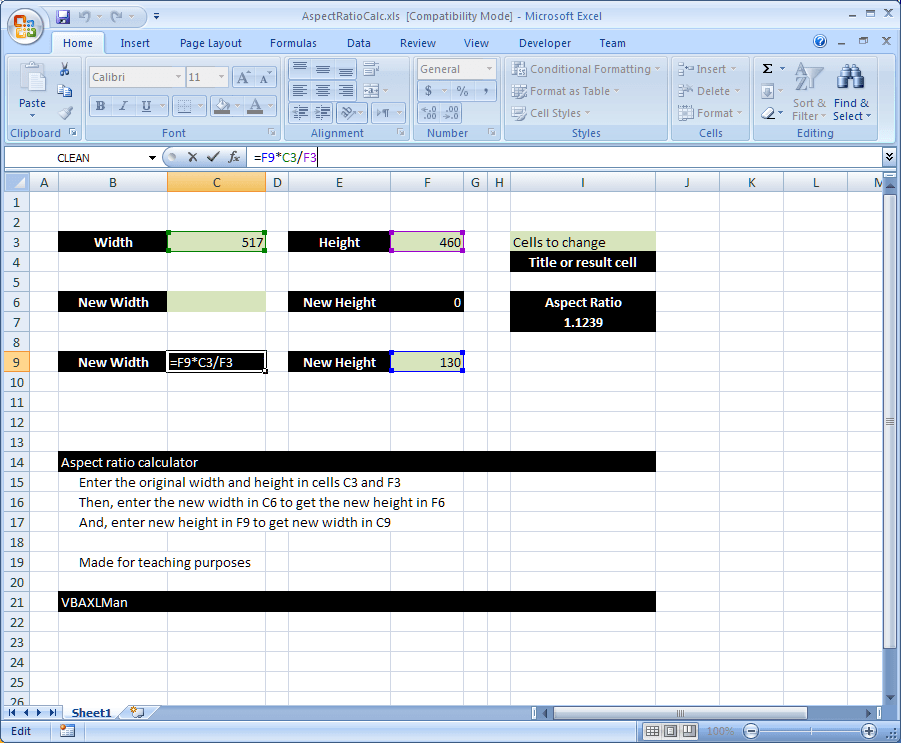Progress bar in pure functionsThis one is very similar to Tally chart, however, it is for another purpose. It is used to draw a progress bar, yes a progress bar in pure functions, using …

Excel Dynamic Drop-Downs that grow with youThis will help you do a dynamic name to use on a drop down, a Form Listbox or Form Combobox. The screenshot almost says it all. You need 4 steps: …

Tally chart in functionsUsing a single function can make a graphical view to your number Function is REPT along with IF and INT to show that number in graphical view, formula used here …

CamelCase to Camel CaseHere is a good practice for combining functions to get a full smartsheet It basically add a space before any capital letter in a text, helpful when you have strings …

Sum of divide – Array formulaThis is the answer to an old question: Here is the story behind it: I had a client who calls me every now and then asking the same question: ” …

Standard math to calculate Aspect RatioHere is a simple formula that uses standard math functions (+ , – , / and *) to calculate the aspect ratio I often need some simple formula like this …